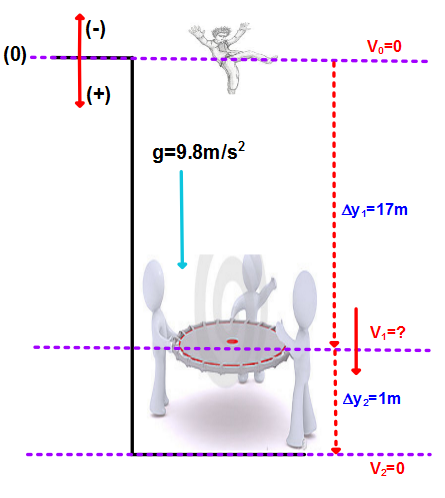×
Get Full Access to Physics: Principles With Applications - 6 Edition - Chapter 2 - Problem 58gp
Get Full Access to Physics: Principles With Applications - 6 Edition - Chapter 2 - Problem 58gp

×

# A person jumps out a fourth-story window 18.0 m | Ch 2 - 58GPISBN: 9780130606204 3

## Solution for problem 58GP Chapter 2

Physics: Principles with Applications | 6th Edition

• Textbook Solutions
• 2901 Step-by-step solutions solved by professors and subject experts
• Get 24/7 help from StudySoup virtual teaching assistantsPhysics: Principles with Applications | 6th Edition

4 5 1 418 Reviews
15
4
Problem 58GP

The acceleration due to gravity on the Moon is about one sixth what it is on Earth. If an object is thrown vertically upward on the Moon, how many times higher will it go than it would on Earth, assuming the same initial velocity?

Step-by-Step Solution:

Step 1 of 3The man jumps from 18m above the ground. The speed of the man increases till he touches the net which is held 1m above the ground. When the man hits the net, he starts to slow down and stops when the net touches the ground.

V0=0

?y1=17m  (the displacement to the net)

?y2=1m    (the height of the net to the ground)

V1=?          (the velocity of the man when he hits the net)

V2=0          (the final velocity of the net when he hits the ground)

a=?           (average acceleration while the net is slowing down the men)

a)

Step 2 of 3

Step 3 of 3

##### ISBN: 9780130606204

This full solution covers the following key subjects: Net, Rest, survivor, deceleration, Person. This expansive textbook survival guide covers 35 chapters, and 3914 solutions. This textbook survival guide was created for the textbook: Physics: Principles with Applications, edition: 6. The full step-by-step solution to problem: 58GP from chapter: 2 was answered by , our top Physics solution expert on 03/03/17, 03:53PM. Physics: Principles with Applications was written by and is associated to the ISBN: 9780130606204. Since the solution to 58GP from 2 chapter was answered, more than 1278 students have viewed the full step-by-step answer. The answer to “?The acceleration due to gravity on the Moon is about one sixth what it is on Earth. If an object is thrown vertically upward on the Moon, how many times higher will it go than it would on Earth, assuming the same initial velocity?” is broken down into a number of easy to follow steps, and 44 words.

Unlock Textbook Solution